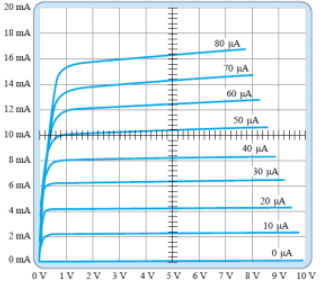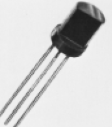Courses

# Test: Limits Of Operation

## 10 Questions MCQ Test Analog Electronics | Test: Limits Of Operation

Description
This mock test of Test: Limits Of Operation for Electrical Engineering (EE) helps you for every Electrical Engineering (EE) entrance exam. This contains 10 Multiple Choice Questions for Electrical Engineering (EE) Test: Limits Of Operation (mcq) to study with solutions a complete question bank. The solved questions answers in this Test: Limits Of Operation quiz give you a good mix of easy questions and tough questions. Electrical Engineering (EE) students definitely take this Test: Limits Of Operation exercise for a better result in the exam. You can find other Test: Limits Of Operation extra questions, long questions & short questions for Electrical Engineering (EE) on EduRev as well by searching above.
QUESTION: 1

### For a BJT, what is typically the shape of the power dissipation curve, if it’s plotted on the output characterisics?

Solution:

Power Dissipation in a BJT is given by P=VCE.IC. This is in the form of k=xy which is the equation of a hyperbola.

QUESTION: 2

### What is the region on the output characteristics below IC = ICEO line called?

Solution:

The region below IC = ICEO is called the cutoff region.

QUESTION: 3

### What is the region on the output characteristics for VCE < VCEsat called?

Solution:

The region below VCE < VCEsat is called the saturation region.

QUESTION: 4

In a BJT amplifier the power gain from base to collector is 4000. The power gain in dB is

Solution:

Gain in dB = 10 log (4000).

QUESTION: 5

Given that the collector power dissipation is 300 mW, what is the value of collector current for the collector to emitter voltage = 12 V?

Solution:

P=VCE.IC => 300mW = (12V)IC => IC=300/12 mA = 25 mA.

QUESTION: 6

Given that the collector power dissipation is 300 mW, what is the value of collector to emitter voltage for collector current = 50 mA?

Solution:

P=VCE.IC => 300mW = VCE(50 mA) => VCE = 300/50 = 6 V.

QUESTION: 7

From the given curve tracer response, what is the value of β for IC = 7 mA and VCE = 5 V?Solution:

From the curve, we get change in IC = (8.2-6.4) mA and change in IB = 10 uA. Hence, /beta; = (1.8/0.01) = 180.

QUESTION: 8

If the positive lead of a DMM, with the mode set to ohmmeter is connected to the base and the negative lead to the emitter and a low resistance reading is obtained, then what is the type of transistor that is being tested?

Solution:

If the positive lead of a DMM, with the mode set to ohmmeter is connected to the base and the negative lead to the emitter and a low resistance reading is obtained, then what is the type of transistor that is being tested is npn.

QUESTION: 9

If the positive lead of a DMM, with the mode set to ohmmeter is connected to the base and the negative lead to the emitter and a high resistance reading is obtained, then what is the type of transistor that is being tested?

Solution:

If the positive lead of a DMM, with the mode set to ohmmeter is connected to the base and the negative lead to the emitter and a low resistance reading is obtained, then what is the type of transistor that is being tested is npn.

QUESTION: 10

For the given transistor, what is the correct sequence of the pins from left to right?Solution:

With the curved side facing away the correct lead sequence is base, collector, emitter.